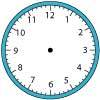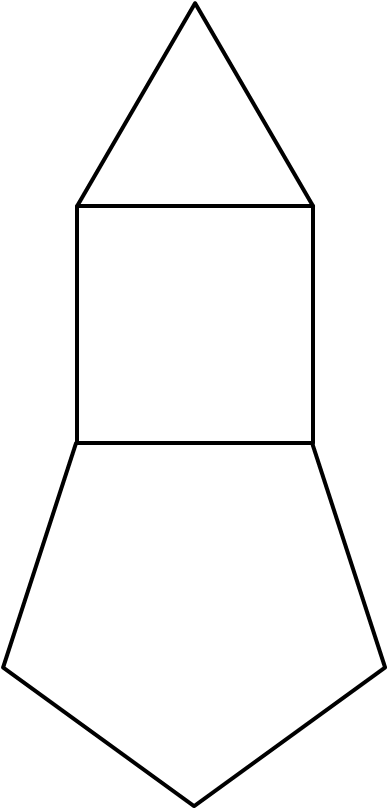#### You may also like### Right Time

At the time of writing the hour and minute hands of my clock are at right angles. How long will it be before they are at right angles again?### Isosceles

Prove that a triangle with sides of length 5, 5 and 6 has the same area as a triangle with sides of length 5, 5 and 8. Find other pairs of non-congruent isosceles triangles which have equal areas.Four rods, two of length a and two of length b, are linked to form a kite. The linkage is moveable so that the angles change. What is the maximum area of the kite?

# Stacking Shapes

##### Age 11 to 14 Short Challenge Level:An equilateral triangle, square and regular pentagon all have the same side length. The triangle is drawn on the top edge of the square, and the pentagon is drawn on and below the bottom edge of the square. All of the edges match up exactly.

What is the sum of the interior angles of the resulting polygon?

If you liked this problem, here is an NRICH task that challenges you to use similar mathematical ideas.
This problem is taken from the UKMT Mathematical Challenges.
You can find more short problems, arranged by curriculum topic, in our short problems collection.